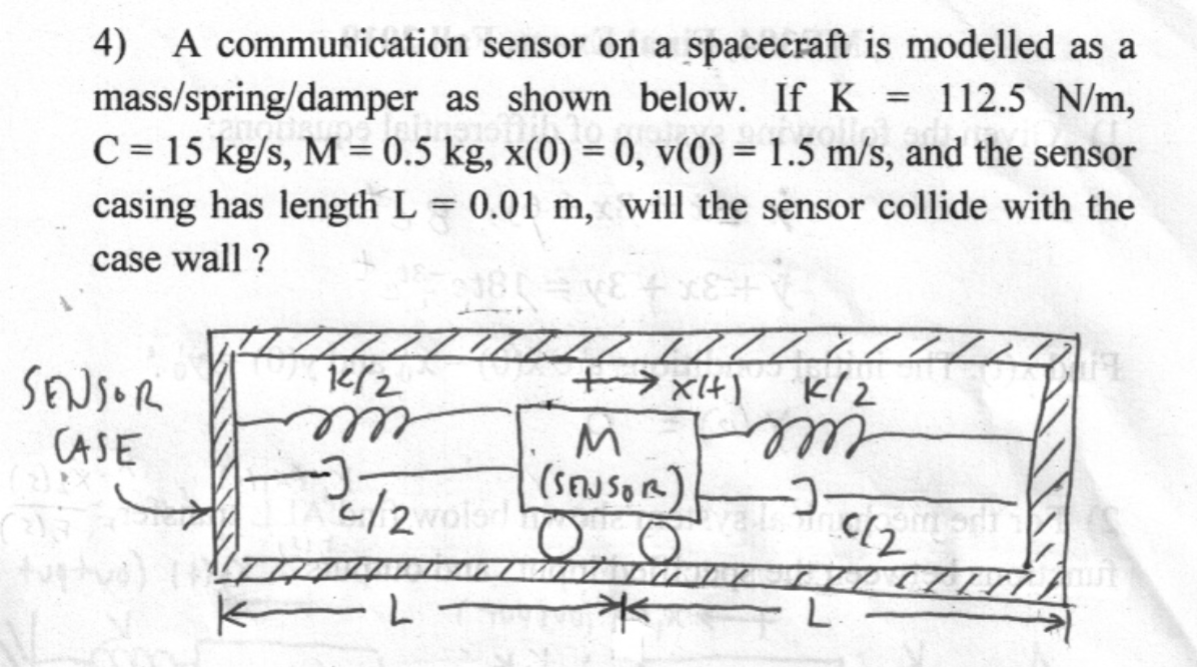Home / Expert Answers / Mechanical Engineering / pls-show-step-by-step-im-really-slow-4-a-communication-sensor-on-a-spacecraft-is-modelled-as-a-ma-pa390

# (Solved): pls show step by step, im really slow 4) A communication sensor on a spacecraft is modelled as a ma ...pls show step by step, im really slow

4) A communication sensor on a spacecraft is modelled as a mass spring damper as shown below. If , , and the sensor casing has length , will the sensor collide with the case wall?

We have an Answer from Expert

To determine whether the sensor will collide with the case wall, we need to solve the differential equation that describes the motion of the mass.

The equation of motion for the mass/spring/damper system is given by:
Mx''(t) + Cx'(t) + Kx(t) = 0
where x(t) is the displacement of the mass from its equilibrium position, M is the mass, C is the damping coefficient, and K is the spring constant.
Substituting the given values, we get:
0.5x''(t) + 15x'(t) + 112.5x(t) = 0
We have an Answer from Expert